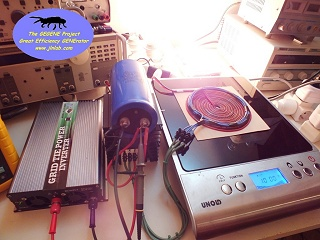TEST #11 : Test of the flat bifilar coil v1.1 with a new induction cooker
created on december 28, 2012 - JLN Labs - last update january 15, 2013
All informations and diagrams are published freely (freeware) and are intended for a private use and a non commercial use.
Toutes les informations et schémas sont publiés gratuitement ( freeware ) et sont destinés à un usage personnel et non commercial

Cliquez ici pour la version FRANCAISE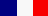January 14, 2013 - TEST #11 : Here is a test of the coil v1.1 with a new induction cooker manufactured by "Rosenstein & Söhne" and with the reference NC-3050. This model of induction cooker is sold for less than 40€ and it is able to give a max power of 1800 Watts and an average power of 1200 W in 10 programmable steps.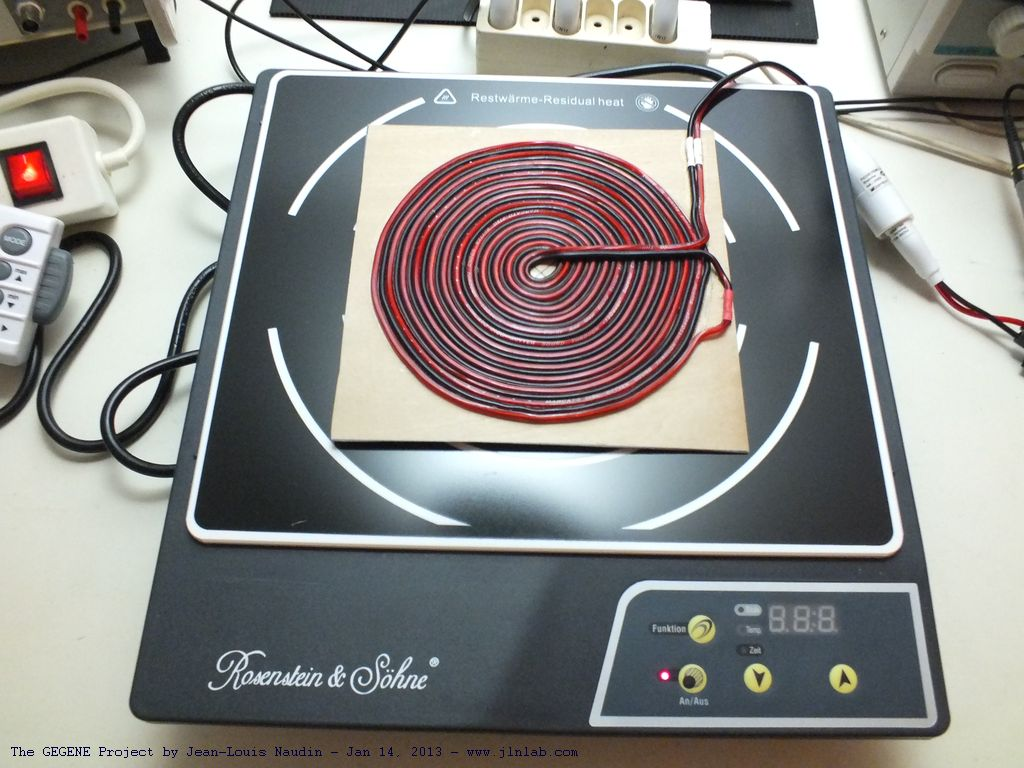Below some photos of the inside of this induction cooker :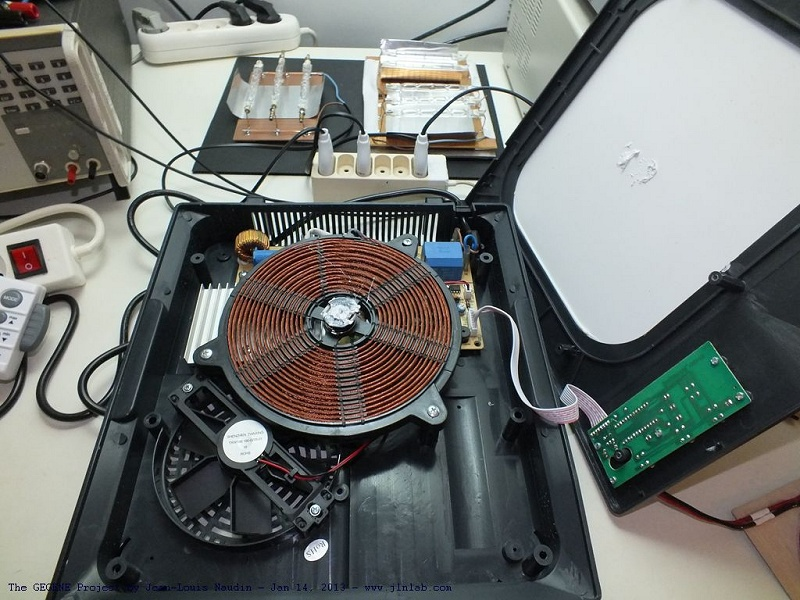The coil of the induction cooker is made with Litz wire, but the wiring setup is a bit different from the previous tested version.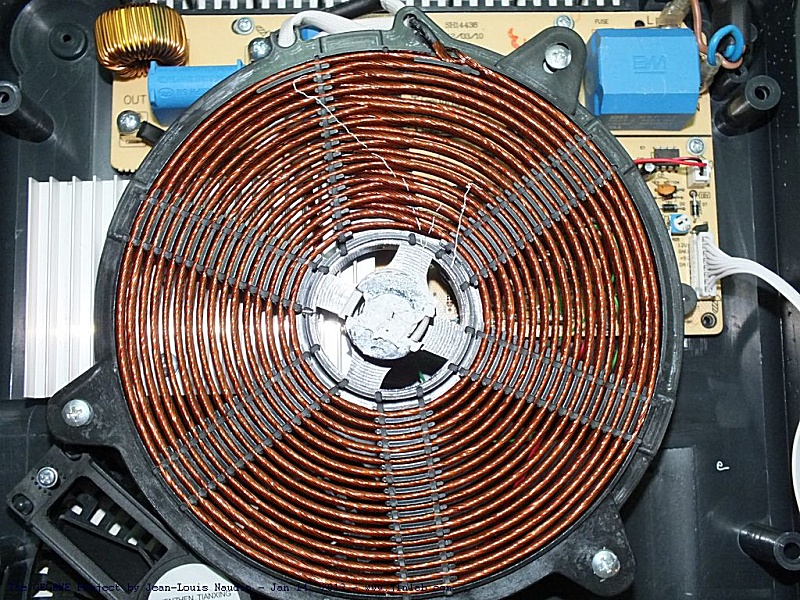The induction coil is made with spaced turns on two interleaved layers...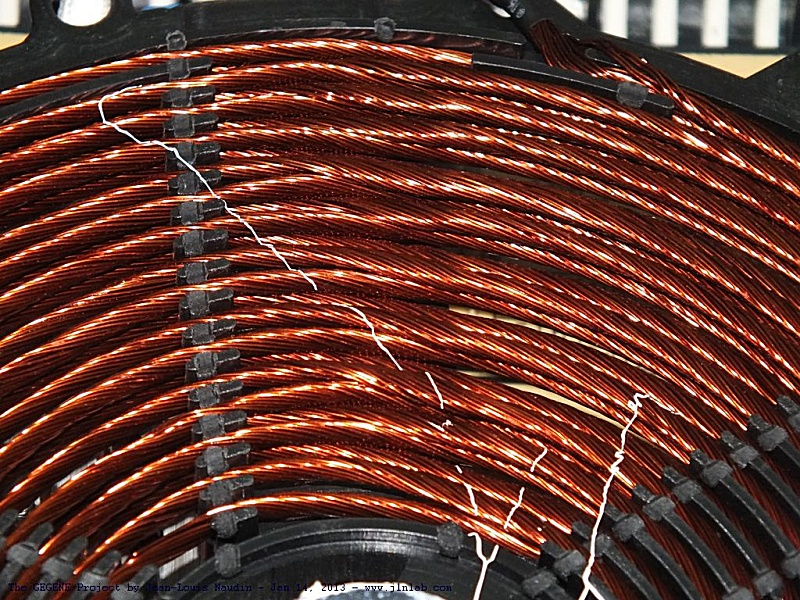Below the electronic control board which drives the induction coil.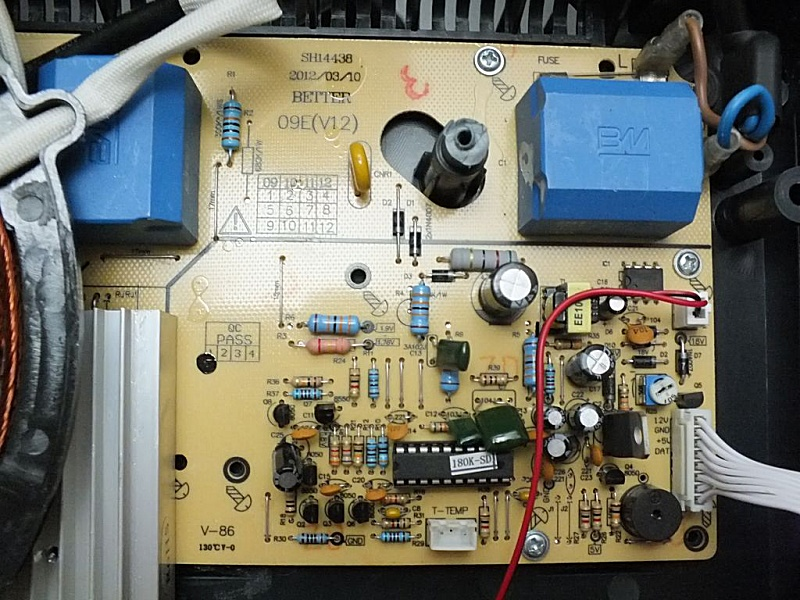To measure the voltage and the current at the output for powering the halogen lamps, the two scope probes are set to X10 and are connected to the flat bifilar coil output. The probe Ch1 is used to measure the voltage accross the flat bifilar coil output and the probe Ch2 is used to measure the current i.e. the voltage accross a 0.1 Ohm non inductive resistor Mundorf MR10 10W. Then the datas are sent to a datasheet to compute true RMS values and the efficiency. The electrical power input of the induction cooker is measured with a Wattmeter Energy Logger 4000F directly connected on the power grid.In this test, I have used the same setup of halogens lamps as for the TEST#5, there are :

• 1 box of 6 halogenes lamps of 105 W
• 2 sets of halogen which contains :
• 1 set of halogens tubes : ((2 x 240 W) + (1 x 400 W)) + (2 x 500 W)
• 1 set of halogens tubes : (1 x 240 W) + (2 x 400 W)

The TOTAL POWER connected at the OUTPUT of the GEGENE is 3550 Watts

Here the configuration of the TEST #11: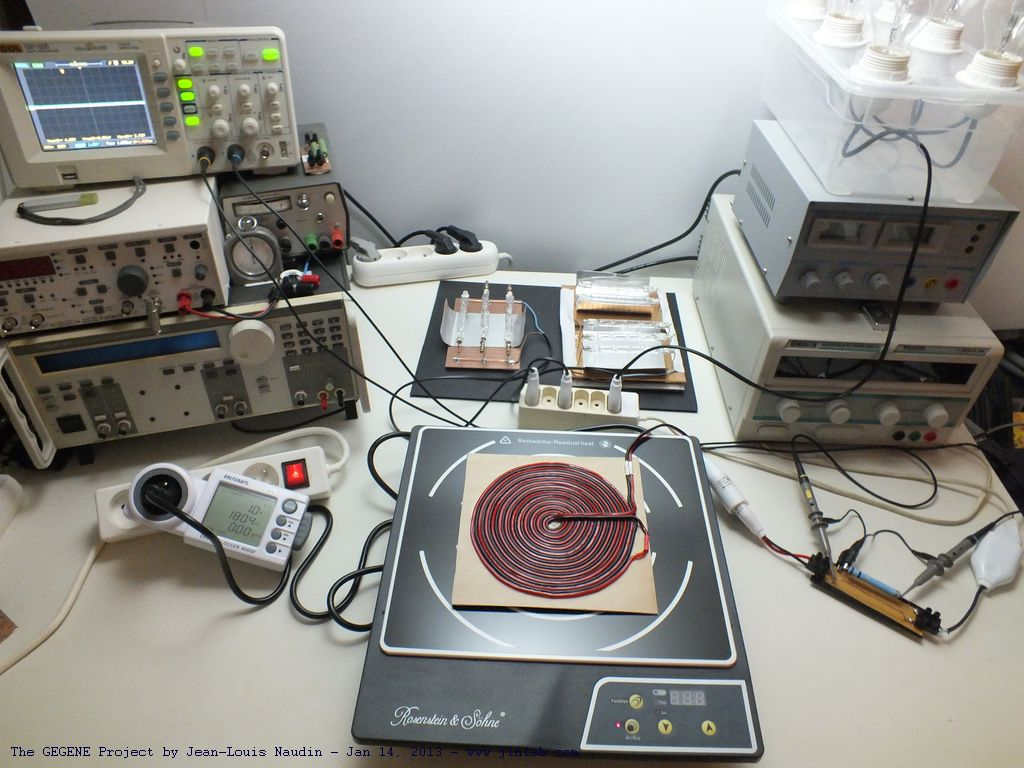TEST #11 RESULTS :

The True RMS values of the voltages on the Ch1 and Ch2 are computed in real time by the Rigol digital oscilloscope ...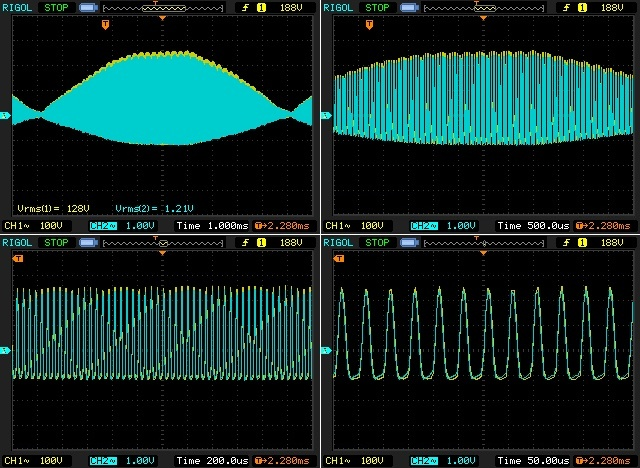Here is an attempt of a mathematical calculation with the Piecewise Cubic Hermite Interpolating Polynomial (PCHIP) method of the digital datas from the oscilloscope, we found :

• U rms integrated on all the datas in Volt (V) with PCHIP:
Trapeze method : 101.822 Volts RMS
Simpson method : 101.544 Volts RMS
• I rms integrated on all the datas in Ampere (A) with PCHIP:
Trapeze method : 10.702 Amperes RMS
Simpson method : 10.671 Amperes RMS
• Average Power integrated on all the datas in Watts (W) with PCHIP:
Trapeze method : 844.482 Watts
Simpson method : 839.802 Watts

Thanks to Pascuser for his interesting work about this mathematical calculation using the PCHIP method for the computation of the digital datas flow coming from the oscilloscope.

The electrical power for the induction cooker is measured with a Wattmeter Energy Logger 4000F directly connected on the power grid :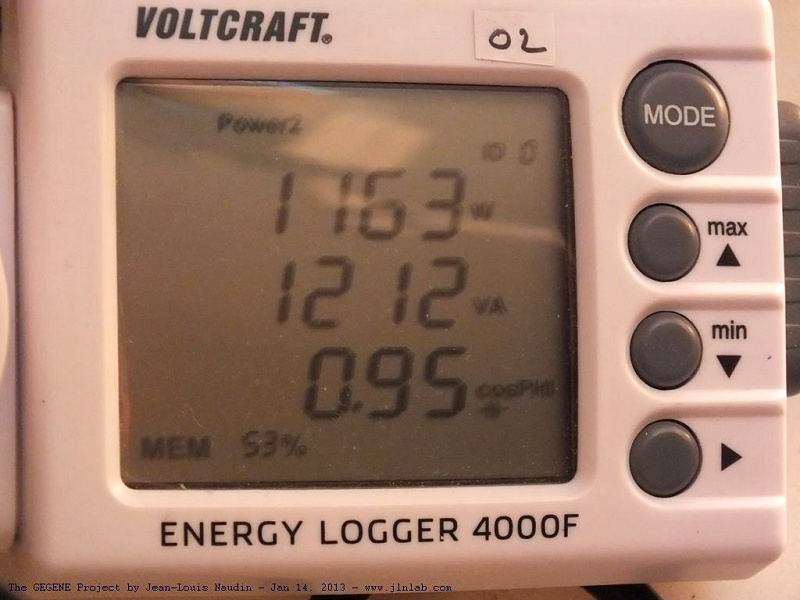The Wattmeter measures 1163 Watt at the INPUT of the induction cooker.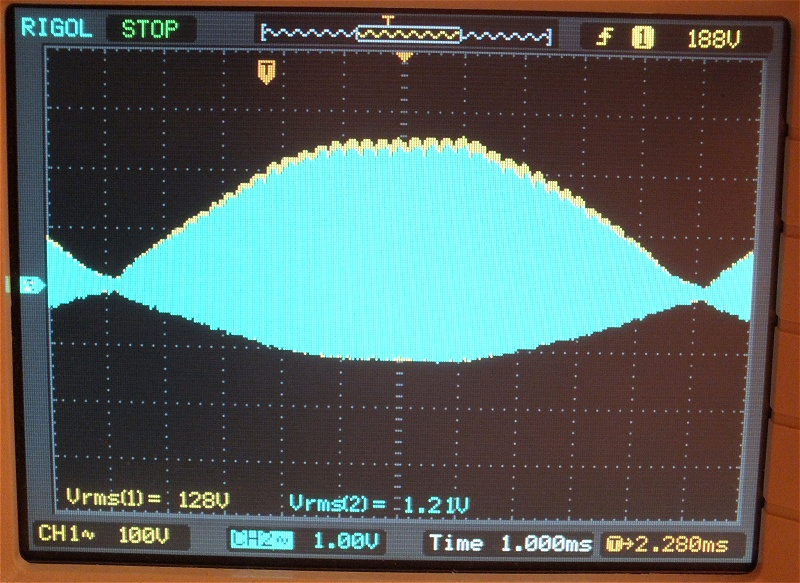Above the True RMS voltage measured by the Rigol digital oscilloscope.

The True RMS voltage measured accross the flat bifilar coil with the probe Ch1 is 128 V rms.
The True RMS voltage measured accross non inductive (metal) resistor of 0.09 Ohm with the probe Ch2 is 1.21 V rms, which gives a measured current of 13.4 A rms.

Comments : We can observe that the mathematical PCIHP method to compute the datas flow gives very different and lower voltages values compared to the True RMS voltages measured in real time by the digital oscillocope. We can also notice that, if we calculate the True RMS values with a datasheet (point to point method) after downloading the datas from the oscilloscope, the RMS values calculated with this method are very close to the the True RMS values calculated in real time with the Rigol digital oscilloscope. Below the results :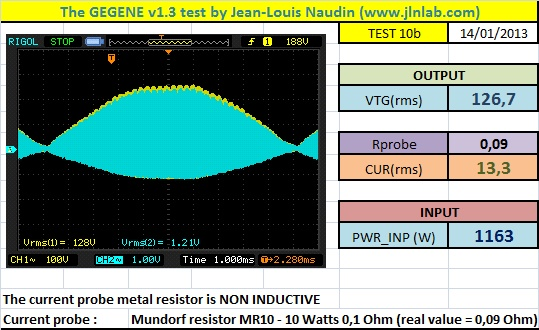True RMS Voltage (Rigol oscilloscope) = 128 V rms, computed RMS voltage (datasheet) = 126.7 V rms,
True RMS Current (Rigol oscilloscope) = 13.4 A rms, computed RMS current (datasheet) = 13.3 A rms

Below, the scope datas computed with GNU Octave 3.2.4 (freeware and full compatible version of MATLAB)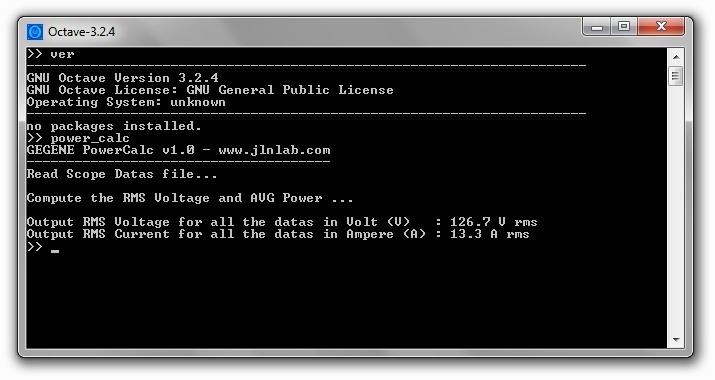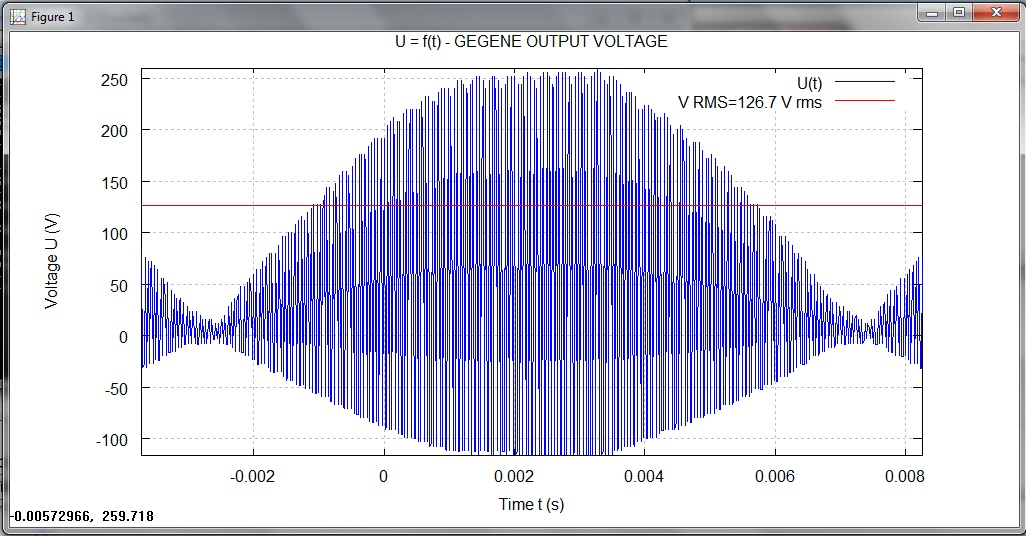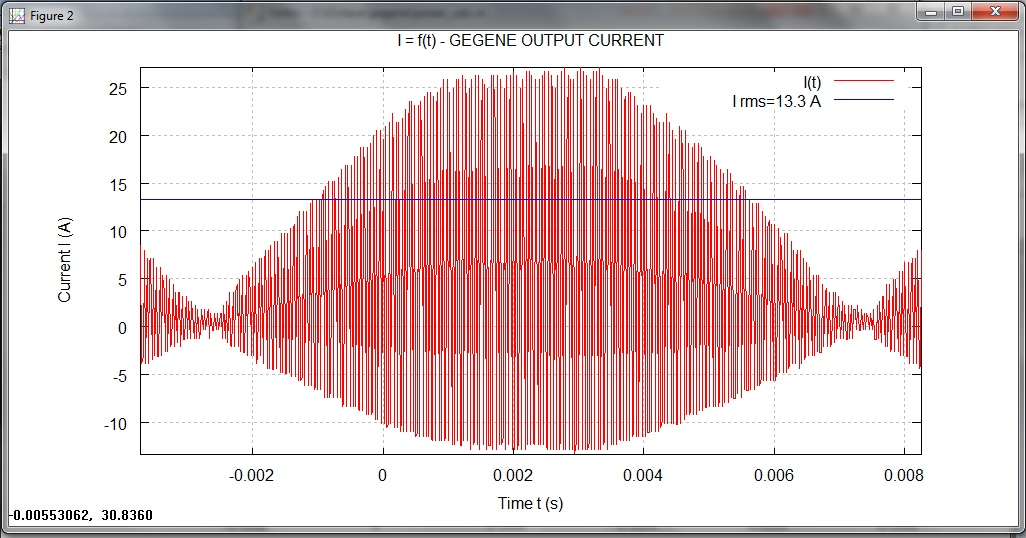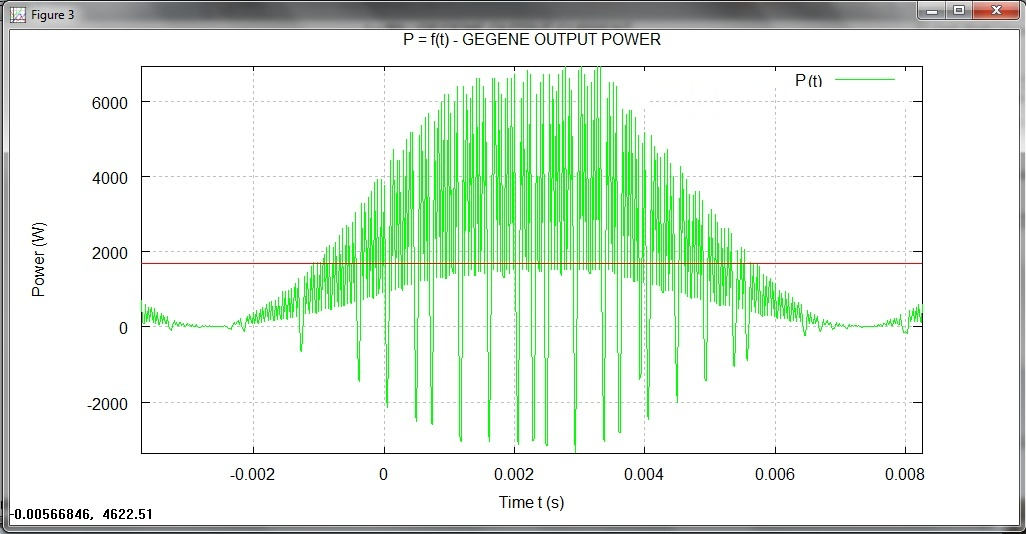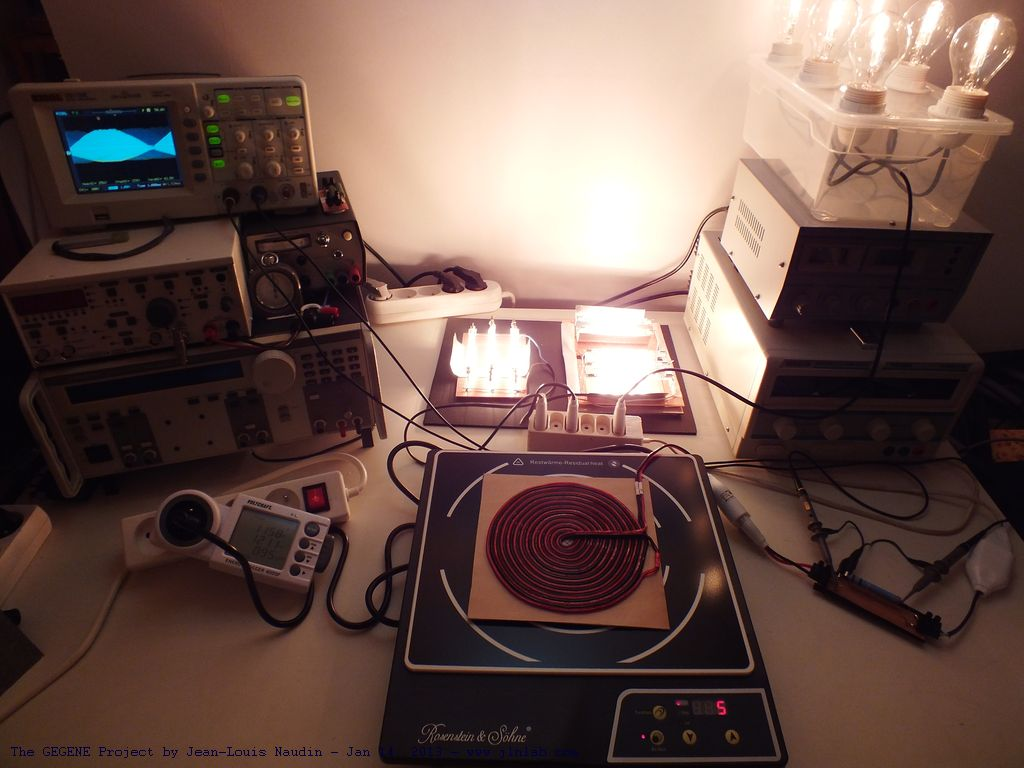With this induction cooker model, I don't need to use a ferromagnetic sheet to start the ignition of the halogen lamps.

NEXT TEST :

Stay tuned,

Email: jnaudin509@aol.com